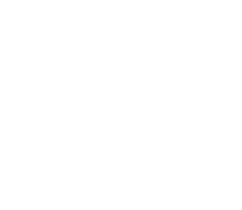# Curriculum

FIFTH YEAR PLAN

FIRST TERM

• Safety in the Laboratory
• Scientific Method
• Geometrical Optics
• Reflection of Light from Spherical Mirrors
• Refraction
• Lenses
• Speed, Displacement and Velocity
• Acceleration
• Vectors and Scalars

SECOND TERM

• Force, Mass and Momentum
• Pressure, Gravity and Moments
• Temperature and Thermometers
• Quantity of Heat transfer
• Work Energy and Power

THIRD TERM

• Waves and Wave Motion
• Vibrations and Sound
• The Wave Nature of Light
SIXTH YEAR PLAN

FIRST TERM

• Static Electricity
• Potential Difference and Capacitance
• Current and charge
• Electromotive Force and Potential Difference
• Resistance
• Effects of Electric Current and Domestic Circuits
• Semiconductors
• Magnets and Magnetic Fields
• Current in a magnetic Field
SECOND TERM

• Electromagnetic Induction
• The Electron
• The Atom, the Nucleus and Radioactivity
• Fission Fusion and Nuclear Energy
• Option
• Circular Motion
• Simple Harmonic Motion

THIRD TERM

Revision